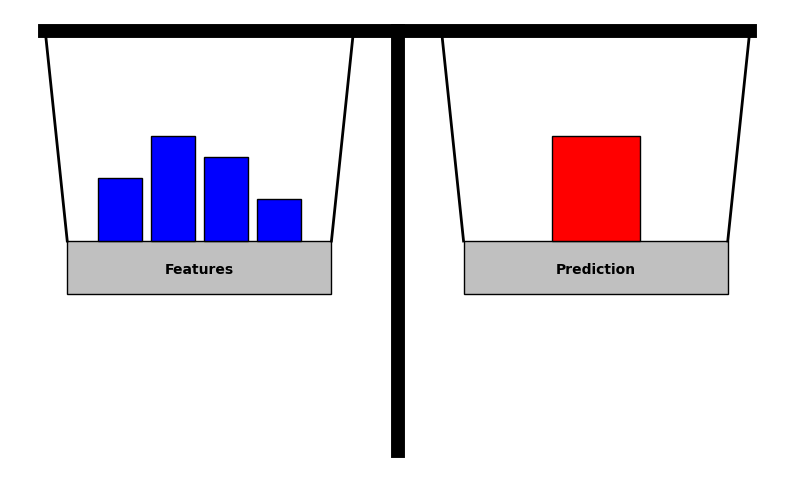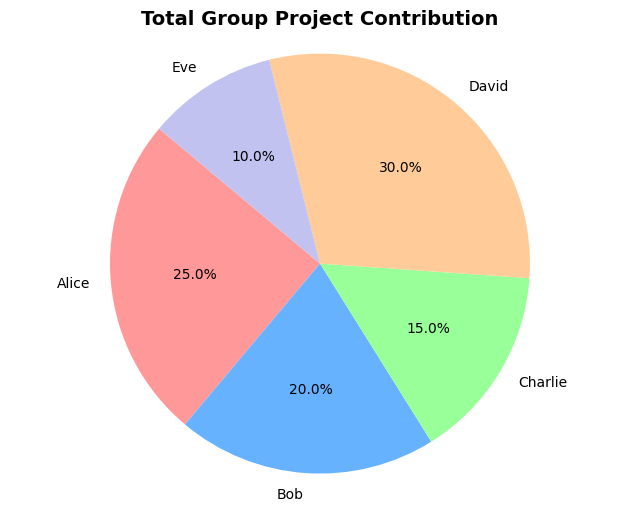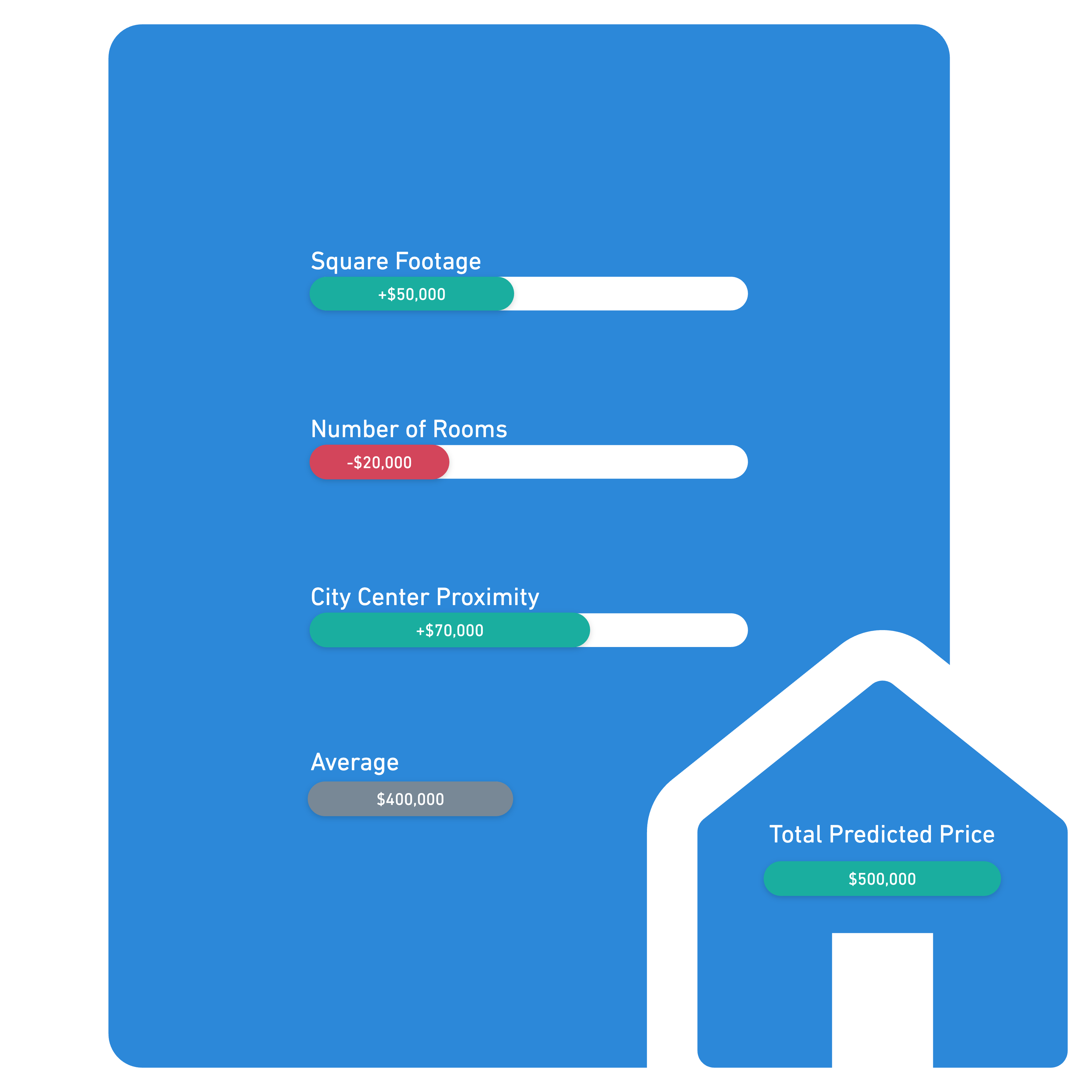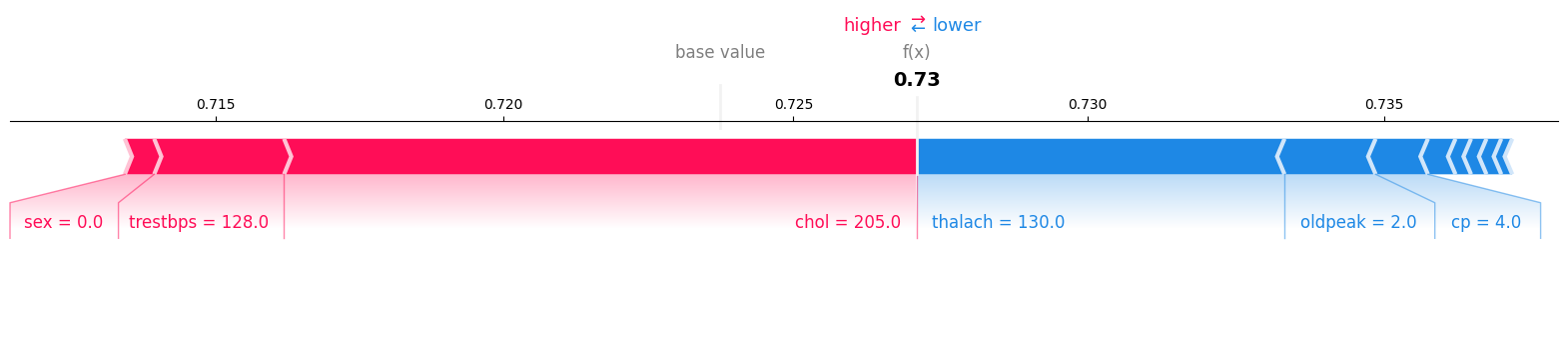Every day, artificial intelligence (AI) and machine learning (ML) technologies are weaving their way deeper into our lives: from healthcare to finance to e-commerce to logistics, these tools are revolutionizing traditional processes, driving innovative solutions, and shaping the speed, precision, and accuracy of how we make business decisions.

As the influence of AI grows exponentially, so too does the demand for transparency. While "black-box" models have often been favored in recent years for their superior performance, the tide is once again shifting. Today, the need for interpretability and explainability is beginning to outweigh the allure of sheer predictive power. Whether it's a patient seeking clarity on a diagnostic algorithm or an investor curious about deeper insights into financial forecasts, there's an increasing necessity to know with confidence how AI systems make their predictions.

Opaque AI models in areas like healthcare, finance, and legal systems can have tangible consequences. A misdiagnosed patient, a denied loan application, or a contentious legal verdict—each instance can erode trust, introduce inefficiencies, and raise ethical alarms. Moreover, these situations bring up questions about the fairness of the AI-driven decisions. Are all factors being considered appropriately? Is any vital aspect being unduly prioritized or ignored? Stakeholders and consumers alike are no longer content with accepting outcomes without insight into the "why" and "how" behind them.Visualizing the equilibrium between individual feature contributions and the model's prediction. Fair attribution balances the scales through transparent understanding to bring peace of mind.

The path to building credibility in AI lies in such transparency -- namely interpretability and explainability. By presenting an AI's reasoning in a comprehensible manner, we can bridge the divide between the highly technical world of ML practitioners and everyone else. At the heart of explainability is the concept of "fair attribution," which ensures each feature or factor gets its due consideration.

In this blog post, we will discuss Shapley Value Regression, a powerful explainability technique that champions this idea of fair attribution to reveals how individual features influence a model's predictions, ensuring a balanced and transparent decision-making process. We will also briefly cover efficient implementations and provide links to relevant resources and tutorials.

## Understanding Shapley Values: Fair Attribution

Before delving into Shapley Value Regression, it's essential to grasp the foundational concept of Shapley values, which underpin this technique. Lloyd Shapley first introduced these values in 1953, not for the world of machine learning, but for cooperative game theory. Picture a game where several players collaborate, and upon completion, there's a collective reward. The challenge lies in fairly allocating this reward based on each player's unique contribution. It becomes even trickier when considering the synergistic effects certain players may have when collaborating.

Shapley values elegantly address this challenge, calculating the marginal contribution of each player across all potential player combinations, ensuring each receives a share commensurate with their overall impact.

In today's context, this concept has been repurposed to demystify the intricate workings of black-box machine learning models. Rather than players in a game, we now examine features in a model. Instead of dividing a game's reward, we ascertain the contribution of each feature to a specific prediction. Each feature's influence is neither undervalued nor overstated, which ensures fairness and offers a more transparent insight into how each feature shapes the model's outcome.A pie chart showing the contribution of each classmate on a school project. If only our teachers had a 'Shapley value' tool to discover those elusive free-riders!

## Comprehending the Formula: How Shapley Values Work And What Actually Makes Them Fair

### The Shapley Value Formula

Given a prediction model, one can think of the "reward" for a particular example as the difference between the prediction for that instance and the average prediction over all instances. This analogy borrows from cooperative game theory, where players share a reward based on their collective effort. Shapley values provide a method to fairly allocate this reward among the model's features, illuminating each feature's contribution.

Mathematically, the Shapley value, $\phi_{i}$, for a feature $i$ is given by:

$$\phi_{i} = \sum_{S \subset N \setminus \{i\}} \frac{|S|!(|N| - |S| - 1)!}{|N|!}(f(S \cup \{i\})-f(S))$$

Where:

• $N$ is the set of all features
• $S$ is any subset of features excluding feature $i$
• $f$ is the prediction model

### Breaking It Down: Shapley Value Formula Explained

• Subsets, $S$, and Features, $N$: The formula evaluates the contribution of feature $i$ by considering all possible subsets $S$ of features that exclude $i$. It looks at how the prediction changes when feature $i$ is added to these subsets.
• Permutations and Factorials: Factorials, represented by the exclamation marks, denote the number of ways a given set of features can be arranged or permuted. The term $\frac{|S|!(|N| - |S| - 1)!}{|N|!}$ calculates the likelihood of a specific subset of features being chosen. Let's break this down further. The numerator computes the permutations for the current subset $S$ and multiplies it by the permutations of the remaining features excluding $i$, which gives us all possible orderings of all features into these three buckets ($S$, $i$, and all other features). The denominator computes the permutations for all features. The ratio ultimately calculates the probability of the particular subset $S$.
• The Difference, $f(S \cup {i}) - f(S)$: This term captures the marginal contribution of feature $i$. It computes the difference in the model's prediction when feature $i$ is included versus when it's excluded. It's worth noting that we could determine the model's prediction for a subset of features by integrating over each feature not in the set; however, we will skip the fine details here since none of the solutions we will discuss actually fully implement this integral.

The Shapley value formula computes a weighted average of the marginal contribution of feature $i$ over all subsets of features weighted by the probability of that subset.

### Desirable Properties: What Makes Shapley Values a "Fair" Distribution

Shapley values are the only attribution method that have the following desirable properties:

• Efficiency: The contributions of all features sum up to the total difference between the prediction and the average prediction. No contribution is left unallocated. Note that this is different from computational efficiency.
• Symmetry: If two features contribute equally to all possible predictions, their Shapley values will be the same.
• Linearity: For two prediction models, the Shapley value of a feature in their combined model is the sum of its Shapley values in each model.
• Stand-alone Test (or Dummy Player): If a feature, when considered alone, does not bring any value (i.e. its sole contribution to the prediction is equivalent to the average prediction or does not change the outcome), then its Shapley value is zero. This ensures that features that don't provide any unique information aren't given undue credit.
• Null Player: If adding a feature to a subset of features does not change the prediction model's output for all subsets, its Shapley value is zero.
• Anonymity: Features are treated based solely on their contributions, not their labels or ordering.
• Marginalism: A feature's Shapley value reflects its marginal contribution on average. This means that the Shapley value is computed based on the additional value a feature provides every time its added to a subset of other features. Features that tend to enhance the model's predictions when included, regardless of the other features present, will have higher Shapley values.

These properties ensure that Shapley values are a meaningful and fair way to attribute feature contributions to the model's prediction.

### Synergy In Shapley Values And Their Implications

In many real-world scenarios, the combined effect of two or more features on a model's prediction is not merely the sum of their individual effects. Imagine two features, $A$ and $B$. Individually they might have a small or even negligible impact on a model's prediction, but the prediction might drastically change when both are present. This combined effect is the synergy between features $A$ and $B$.

The Shapley formula does not just consider individual feature contributions; it assesses all possible combinations of features. This means that it inherently captures these synergistic effects. When computing the Shapley value for feature $A$, the formula evaluates scenarios both with and without feature $B$ (and vice-versa). This comprehensive evaluation ensures that the contribution of $A$ accounts for its synergy with $B$ and all other features. So what are the implications of accounting for this synergy?

• Complex Interactions: Real-world data often contains intricate relationships. Two features might be redundant when considered separately but highly informative when combined. Shapley values help uncover such interactions.
• Fair Attribution: By accounting for synergy, Shapley values prevent the underestimation or overestimation of a feature's contribution. This ensures each feature is credited fairly based on both its individual and synergistic impacts.
• Model Improvements: Recognizing synergistic interactions can provide insights into the data and the model. It can guide feature engineering efforts and even lead to better model architectures.

## Shapley Value Regression: Calculating Shapley Values

Shapley Value Regression involves a direct computation of Shapley values using the formula we discussed earlier. To recap, the Shapley value $\phi_{i}$ is given by:

$$\phi_{i} = \sum_{S \subset N \setminus \{i\}} \frac{|S|!(|N| - |S| - 1)!}{|N|!}(f(S \cup \{i\})-f(S))$$

Breaking down the formula:

• The term $\frac{|S|!(|N| - |S| - 1)!}{|N|!}$ calculates the likelihood of a specific subset of features $S$. This is straightforward to compute.
• The challenge lies in determining the difference $f(S \cup {i}) - f(S)$. If we have a model trained on the complete set of features, how can we obtain predictions using only subsets of these features?

One direct solution is to train individual models for each possible subset of features. However, this requires training $2^{|N|}$ separate models. As the number of features increases, this approach quickly becomes computationally demanding and, in some cases, practically infeasible.

So, the question is: how can we more efficiently compute Shapley values?

### Monte Carlo Estimation: Making Shapley Value Regression Feasible

Instead of training multiple models, we can use Monte Carlo estimation with just one model to approximate the Shapley values. The idea is to randomly sample feature values to estimate the contribution of each feature.

Imagine a model with features $x = (x_{1},...,x_{i},...x_{n})$. For a subset $S$, we retain the original values for the features in $S$ and replace all other features not in $S$ with random values. This gives a (very) rough estimate of the prediction $f(S)$. We can do the same for $f(S \cup {i})$, calculate the difference, and average over many samples to refine our estimate.

To further improve the estimate, we can use random permutations of features. For each permutation, we calculate the prediction using the original values up to feature $i$ in the permutation and replace all subsequent values. By averaging over many permutations, we approach the true Shapley value.

In practice, we use the following formula:

$$\hat{\phi}_{i} = \frac{1}{M} \sum_{m=1}^{M} (P_{\pi_m}(i) - P_{\pi_m}(i-1))$$

Where:

• $\hat{\phi}_{i}$ is the estimated Shapley value for feature $i$
• $M$ is the number of random permutations sampled
• $\pi_{m}$ is the $m$-th random permutation of all features
• $P_{\pi_{m}}(i)$ is the prediction when features up to $i$ in the permutation use their original values, while the rest are replaced with values from a randomly selected sample from the dataset, ensuring realistic values.

## Illustrating Shapley Values with A Practical Example

The beauty of Shapley values lies in their ability to break down complex model predictions into more understandable components. Let's illustrate this with a practical example.

### Predicting House Prices

Imagine we have a dataset of houses and their respective selling prices. We've built a machine learning model that predicts a house's price based on the following three features: square footage, number of rooms, and proximity to the city center.

For a specific house, our model predicts a price of $\$500,000$. But how did the model arrive at this prediction, and how much did each feature contribute? Using Shapley values, we can break down the prediction relative to the average predicted price of$\$400,000$:

• Square Footage: If this house has a larger square footage than most houses in the dataset, this feature might significantly increase its predicted price. Let's say the Shapley value for square footage is $\$50,000$. This means that the size of the house raises its price by$\$50,000$ above the average.
• Number of Rooms: Suppose our house has an average number of rooms. The Shapley value might be neutral or even slightly negative. Let's assume the Shapley value for the number of rooms is $-\$20,000$. This indicates that the number of rooms reduced the predicted price by$\$20,000$ below the average, perhaps because other houses with a similar number of rooms in the dataset generally have a low price.
• Proximity to the City Center: Being close to the city center is often a desired feature for many homebuyers. If our house is particularly close to the city, this could raise its predicted price. The Shapley value might be $\$70,000$, suggesting an increase due to its prime location. Adding up the average predicted price and the Shapley values, we get:$\$400,000$ (average) $+$ $\$50,000$(square footage)$-\$20,000$ (number of rooms) $+$ $\$70,000$(proximity)$=\$500,000$, which matches our model's prediction.A breakdown of feature contributions to a house's predicted price, showcasing the power of Shapley values.

The process of using Shapley values guarantees that each feature's influence is transparent. By allocating the predicted price among the features, we can see the "fair" contribution of each feature. In our example, the square footage and proximity to the city center had positive contributions, while the number of rooms slightly decreased the predicted price. This kind of breakdown is invaluable for understanding, validating, and communicating the model's predictions.

## Interpreting Shapley Values: Insights and Applications

The essence of Shapley values lies in their ability to provide a fair allocation of contributions across features. However, their utility stretches beyond explanation. Let's explore some of the key insights and applications that Shapley values offer:

### Identifying Key Features

By analyzing the Shapley values across multiple predictions, we can identify patterns in feature influence. Features with consistently high Shapley values across many instances are likely to be pivotal in the decision-making process of the model. This insight can be crucial when deciding which features to focus on, especially in domains where feature extraction or data collection is costly or time-consuming.

### Refining Models

Shapley values can be a valuable tool in the model refinement process. If a feature consistently has a near-zero Shapley value, it might be a candidate for removal, potentially simplifying the model without sacrificing performance. Conversely, features with high variability in their Shapley values could be further investigated to understand the underlying reasons and improve future model stability.

### Debugging Complex Models

Sometimes, models make unexpected predictions. Shapley values can act as a diagnostic tool in such cases. By breaking down the prediction into feature contributions, we can pinpoint which features led to the unexpected prediction and investigate potential issues, be it data anomalies, leakage, or model biases.

### Ensuring Fairness and Transparency

In sectors like finance or healthcare, where model decisions have significant real-world implications, understanding feature contributions is crucial. Shapley values help ensure that decisions are made more transparently to help in validating the model's decisions for better compliance with regulations. Of course, the values themselves do not guarantee compliance.

### Aiding Stakeholder Communication

Not every stakeholder is well-versed in machine learning intricacies. Shapley values, when visualized effectively, can bridge the communication gap. By showing feature contributions in a digestible format, stakeholders can better understand model decisions, fostering trust and collaboration.

### Enhancing Feature Engineering

By Understanding which features are influential and which aren't, data scientists can direct their feature engineering efforts more effectively. If a newly engineered feature consistently shows high Shapley values, it's an indication that the feature is adding valuable information to the model.

## Shapley Values vs. Other Interpretability Techniques

There's no shortage of techniques aiming to make AI transparent. However, where Shapley values shine is in their ability to offer consistent and fair attributions, capturing intricate feature interactions that other methods might overlook. Here's how Shapley values stack up against some of the other prominent methods:

### Feature Importance

• Definition: Feature importance ranks features based on their impact on the model's output. Techniques like Gini importance or permutation importance are commonly used.
• Advantages: Simple to compute, intuitive, and provides a global view of feature significance.
• Limitations: Doesn't capture feature interactions. Can be misleading if features are correlated.

### Partial Dependence Plots (PDP)

• Definition: PDP shows the effect of a feature on the model's prediction, keeping other features constant.
• Advantages: Visual and intuitive. Helps in understanding feature behavior and its impact on predictions.
• Limitations: Assumes features are independent, which may not always be the case.

### LIME (Local Interpretable Model-agnostic Explanations)

• Definition: LIME approximates a complex model using a simpler, interpretable model for individual predictions.
• Advantages: Model-agnostic and provides interpretable results for individual predictions.
• Limitations: Local explanations might not always generalize. The choice of the simpler model and features might impact results.

### Counterfactual Explanations

• Definition: Explains model decisions by showcasing what would need to change in the input to get a different prediction.
• Advantages: Intuitive and actionable. Helps in understanding decision boundaries.
• Limitations: Can be computationally intensive. Doesn't always provide a clear picture of feature influence.

While these explainability techniques offer valuable insights, Shapley values stand out for their rigor, fairness, and ability to capture nuanced feature interactions. They provide a robust and comprehensive framework for understanding model decisions, making them a favored choice in many interpretability scenarios. However, Shapley values are by no means perfect.

## Challenges and Considerations: When to Use Shapley Values

### Computational Complexity

• Challenge: The direct computation of Shapley values using traditional Shapley value regression involves evaluating the model's prediction for every possible combination of features. This can be computationally expensive, especially as the number of features grows.
• Consideration: Before choosing Shapley values, consider the number of features in your model and the computational resources available. Approximate methods like the Monte Carlo estimation can be used to mitigate this challenge.

• Challenge: While Shapley values provide detailed insights, they might be overkill for tasks where a simpler, high-level understanding works as well to get the insights needed.
• Consideration: Evaluate the depth of interpretation required. If a general sense of feature importance is adequate, simpler methods might be more appropriate.

### Model Type and Complexity

• Challenge: For some complex models, like deep neural networks (DNN), the explainability using Shapley values can be intricate and less intuitive.
• Consideration: For simpler models like linear regression or decision trees, other interpretability techniques might be more straightforward. However, for ensemble methods or black-box models, Shapley values offer a level of insight that's hard to match.

### Real-time Requirements

• Challenge: Due to their computational demands, Shapley values might not be suitable for applications requiring real-time explanations.
• Consideration: In scenarios where instant interpretability is needed, consider faster, albeit potentially less detailed, methods.

### Synergy and Interaction Effects

• Challenge: Not all interpretability techniques can capture the synergistic effects of features effectively.
• Consideration: If understanding feature interactions is crucial, Shapley values are one of the best techniques available, as they evaluate all possible feature coalitions.

### Correlated Features

• Challenge: Shapley values can include unrealistic instances during the random sampling process when features are correlated since the replaced value may not be realistic given the retained value of the correlated feature.
• Consideration: Before interpreting Shapley values, it's crucial to be aware of the correlations between features in the dataset. If features are highly correlated, their individual Shapley values should be interpreted with caution.

### When to Use Shapley Values?

Given their strengths and challenges, here are scenarios where Shapley values shine:

1. High-Stakes Decisions: In fields like healthcare or finance, where decisions have significant consequences, the comprehensive insights from Shapley values can be invaluable.
2. Regulatory Compliance: For industries subject to stringent regulations around model transparency, Shapley values offer a rigorous and accepted method for model explanations.
3. Debugging Complex Models: When trying to understand unexpected model behavior, Shapley values can help identify features that are contributing disproportionately.
4. Feature Engineering: By understanding feature contributions, data scientists can refine and optimize features for better model performance.

## Tools and Libraries: Implementing Shapley Value Regression in Practice

For those eager to delve deeper, you can check out some libraries and tutorials for common ways of computing Shapley values in practice.

### SHAP (SHapley Additive exPlanations) Library

One of the most popular libraries for computing Shapley values for machine learning models. Developed by Scott Lundberg and his team, SHAP provides a unified measure of feature importance by leveraging the concepts we've discussed. The library supports a wide range of models and offers various visualization tools to interpret the results.

#### Key Features

• TreeExplainer: Optimized for tree-based models like XGBoost, RandomForest, and LightGBM.
• DeepExplainer: Designed for deep learning models, particularly neural networks.
• KernelExplainer: A model-agnostic method to estimate SHAP for any model.
• LinearExplainer: Ideal for linear models.
• Visualizations: Offers plots like force plots, summary plots, and dependence plots to visualize feature importance.A force chart from the SHAP library showing the impact of each feature on the prediction for a particular example. In this case, the model is trying to predict heart disease based on various medical factors. This is just one of the available chart types.

## Other Libraries and Tools

• InterpretML: An open-source library for interpreting machine learning models. It offers various methods, including Shapley values, to help in model explanations.
• FairML: Focuses on deciphering the black-box predictive models, ensuring they are fair and free from biases. It uses concepts similar to Shapley values to understand feature importances.
• LIME (Local Interpretable Model-agnostic Explanations): While not strictly focused on Shapley values, LIME is another popular tool in the model interpretability arsenal. It offers explanations by approximating black-box models with interpretable models.

## Conclusion: Empowering Explainable AI

Shapley values currently stand at the forefront of the move towards transparent and explainable AI. By ensuring fair feature attribution, they not only make models understandable but also build trust in AI systems. As we continue to integrate AI into our lives, the call for clear, interpretable models will only grow louder. Shapley values, with their promise of fairness and clarity, are one of the current answers to that call.

## References

https://www.rand.org/content/dam/rand/pubs/research_memoranda/2008/RM670.pdf

Shapley, L. S.. "17. A Value for n-Person Games". Contributions to the Theory of Games (AM-28), Volume II, edited by Harold William Kuhn and Albert William Tucker, Princeton: Princeton University Press, 1953, pp. 307-318. https://doi.org/10.1515/9781400881970-018

Štrumbelj, Erik and Igor Kononenko. “Explaining prediction models and individual predictions with feature contributions.” Knowledge and Information Systems 41 (2014): 647-665.

https://christophm.github.io/interpretable-ml-book/shapley.html#fn64